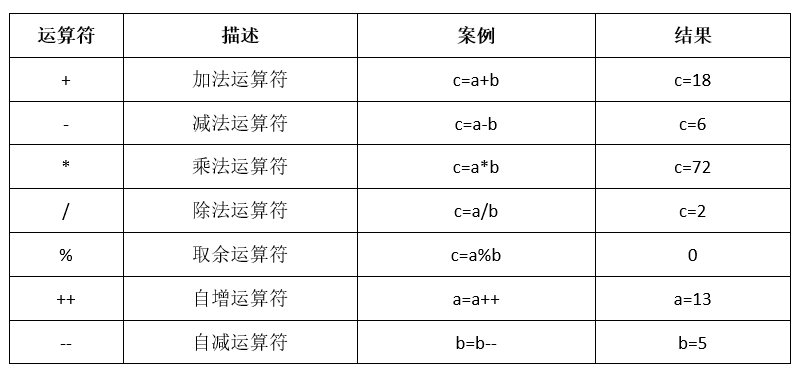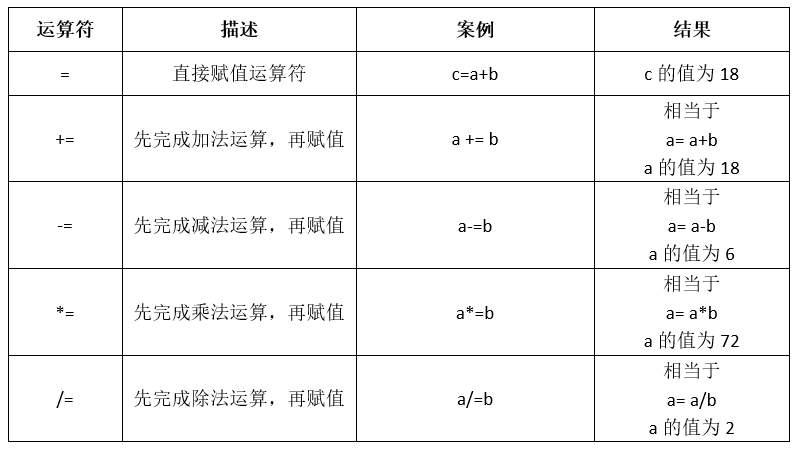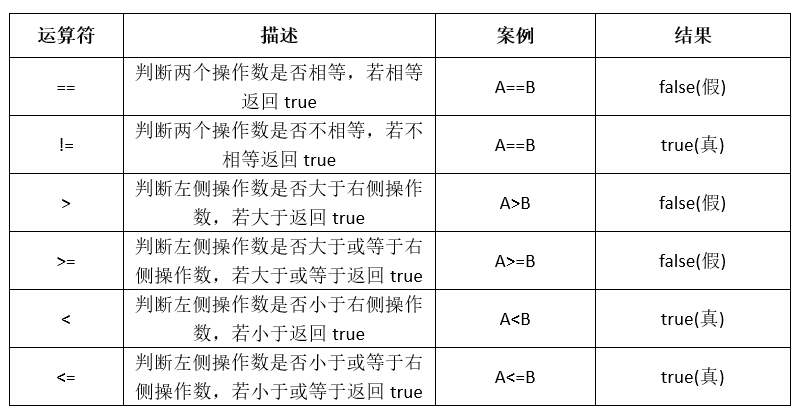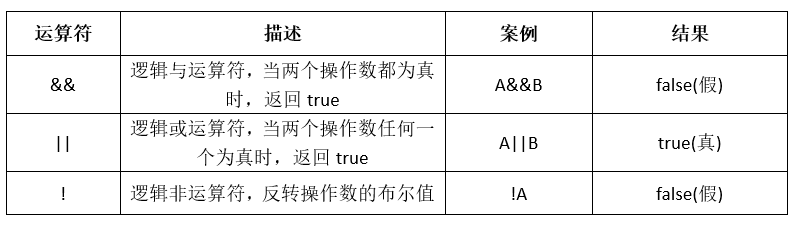JavaScript基本语法
3210字，阅读需时11分钟

JavaScript语法与Java语法类似，但JavaScript的语法规则要比Java语言的语法规则简单的多。

1、语句

JS语句同Java语句相同，在语句中可以包含变量、关键字、运算符和表达式，语句结束符使用英文分号“；”，在语句的结尾也可以不使用结束符。

```name = "赵五";
r = 3.0;
s = 3.14 * r * r;```

```<html>
<title>这是登录页面</title>
<meta http-equiv="Content-Type" content="text/html; charset=utf-8" />
<script type="text/javascript">
function verify(){
name = "赵五";
r = 3.0;
s = 3.14 * r * r;
}
</script>
<body>
<h3>JS语句案例代码1</h3>
<hr>
<p><input type="button" value="计算圆的面积" onclick="verify()"/></p>
</form>
</boyd>
<html>```

2、  代码块

JS代码块使用一对大括号“{}”将多条JS语句组合在一起，完成一个特定的功能。JS代码块一般在函数、条件结构、循环结构内部使用。

```function verify(){
name = "赵五";
r = 3.0;
s = 3.14 * r * r;
}```

3、  标识符与变量

JS标识符和Java标识符相同，都是用于标识变量、函数、关键字、类等名称。标识符只能有字母、数字和下划线组成，不能包含其它符号。标识符的第一个字符必须是字母、下划线，不能是数字。

```name
Age
Num1```

JS内部预定义了一些标识符，这些标识符是JS关键字，它们不能用于变量、函数和类的命名。JavaScript关键字见下表：`r = 3.0`

`var r = 3.0`

`const PI = 3.14`

4、  注释

JavaScript注释同Java注释基本相同，注释也分为单行注释和多行注释两种。

```// 声明圆周率PI
const PI = 3.14;```

“// 声明圆周率PI”就是注释语句，注释语句前面使用“//”开始，该注释语句对代码const PI = 3.14进行了解释。

`const PI = 3.14;   // 声明圆周率PI`

```/*
函数名称：area

日期：2020-10-08
*/
function area(){
r = 3.0;
s = 3.14 * r * r;
}```

5、  运算符

JavaScript运算符与Java运算符基本相同，主要包括算术运算符、赋值运算符、关系运算符、逻辑运算符，条件运算符。```a = 10;
b = 3;
value = a>b?a+6:b+6;```

字符串连接运算

```s1=”Hello”;
s2=” JavaScript”
s = s1+s2;